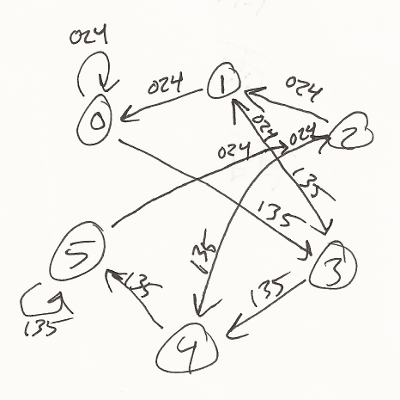# Raincomplex's State Transition Graph Dec 20, 2011

(for context, see everything2)The image in question is a state transition graph I drew (pencil, 5cm x 5cm), with six nodes, transcribed below.

```0 --024--> 0
0 --135--> 3
1 --024--> 0
1 --135--> 3
2 --024--> 1
2 --135--> 4
3 --024--> 1
3 --135--> 4
4 --024--> 2
4 --135--> 5
5 --024--> 2
5 --135--> 5
```

The rules describe a one-dimensional six-state cellular automaton with an asymmetric neighborhood, with one extra state not shown above (called 6). Oops. I mean, I totally omitted it so as to preserve the beautiful symmetry of the graph. The rules for 6 are: 6 --0246--> 6 and 6 --135--> 4. In each rule, the left number is the current cell, the center numbers are allowed values for the cell just to its left, and the right number is the value of the current cell in the next generation.

A state is a finite sequence of cells valued 0-5. To the left of this sequence is an infinite number of 0s, and to its right an infinite number of 6s. (Below I indicate this with a single 0 and 6 on either side of the state.) So suppose we begin with the state 31:

```Generation  1:  0316

03   a 3 with a 0 to the left becomes a 1
1   (3 --024--> 1)

31   a 1 with a 3 to the left becomes a 3
3   (1 --135--> 3)

16   a 6 with a 1 to the left becomes a 4
4   (6 --135--> 4)
so,
Generation  2:  01346
Generation  3:   0456
Generation  4:   02246
Generation  5:   01126
Generation  6:    0346
Generation  7:    0156
Generation  8:     0546
Generation  9:     0256
Generation 10:     01246
Generation 11:      0426
Generation 12:      0216
Generation 13:      01046
Generation 14:       0326
Generation 15:       0146
Generation 16:        056
Generation 17:        0246
Generation 18:        0126
Generation 19:         046
Generation 20:         026
Generation 21:         016
Generation 22:          046
Generation 23:          026
Generation 24:          016
Generation 25:           046
```

You'll notice that the numbers "travel" to the right, eating into the infinite 6s there. At the end, the state begins to cycle (albeit offset by one cell each cycle) between 4, 2, and 1. Does this seem familiar? If we treat the states as integers in base 6, ignoring the 6s:

```Generation  1:  0316 = 19
Generation  2:  01346 = 58
Generation  3:   0456 = 29
Generation  4:   02246 = 88
Generation  5:   01126 = 44
Generation  6:    0346 = 22
Generation  7:    0156 = 11
Generation  8:     0546 = 34
Generation  9:     0256 = 17
Generation 10:     01246 = 52
Generation 11:      0426 = 26
Generation 12:      0216 = 13
Generation 13:      01046 = 40
Generation 14:       0326 = 20
Generation 15:       0146 = 10
Generation 16:        056 = 5
Generation 17:        0246 = 16
Generation 18:        0126 = 8
Generation 19:         046 = 4
Generation 20:         026 = 2
Generation 21:         016 = 1
Generation 22:          046 = 4
Generation 23:          026 = 2
Generation 24:          016 = 1
Generation 25:           046 = 4
```

When decreasing, the numbers are being divided by two (ni+1 = ni/2). When increasing, they are following the formula ni+1 = 3ni+1. This is the hailstone sequence, which if the Collatz conjecture is true, does always end with the (4, 2, 1) cycle, for any initial state ≥ 1.

Why does this work? In short, because 3n = 6(n/2). That is, in base 6, multiplying by three is the same thing as dividing by two and then shifting one digit to the left (i.e., adding a 0 on the right side). The rules at the top of this writeup describe division by two in base 6*. Thus to multiply by three and add one**, we simply need to move everything a digit to the left and add 4***, then divide by two as normal, according to the rules.

* The derivation of which is tedious beyond the scope of this writeup.
** as per the Collatz function when the argument is odd (which in base 6 occurs when it ends in 1, 3, or 5)
*** The rules perform truncating division for odds---adding 4 makes up for both the truncation (1/2 in base 6 is ".3") and the "add one" part of 3n+1. This addition is the sole reason for the extra symbol (6).

Does this get us closer to a solution to the conjecture? Who knows, and probably not. The whole thing is so simple and yet something flabbergastingly impenetrable is happening. It's very easy to restate in other ways, and so far that hasn't helped anybody figure it out.

Raincomplex's State Transition Graph

category: factuals
previous: Designing to Reveal The Nature of the Universe

all writing, chronological
next: Levenshtein dreams
previous: a lie I needed to believe